# Programming Videos

## Search

### Write a Program to Reverse a String Using Stack

Write a C, C++ program to reverse a string using Stack data structure. In this question, A string is input by a user and our program will reverse a string using a Stack.

## Stack Data Structure

Stack is an abstract data type in which push (insertion) and pop (deletion) operations can be done at one end.  Stack is a LIFO (Last in First Out) data structure, which means element which is inserted at last must be the first one to be removed.

Some important articles regarding Stack data structure and it's implementation.

Stack program in C using an Array

Implement Stack data structure

MCQ on stack and queue for practice

Reverse a string using for and while loop

## Program to Reverse a String Using Stack Data Structure

```#include <stdio.h>
#include <string.h>

#define max 100
int top,stack[max];

void push(char x){

// Push(Inserting Element in stack) operation
if(top==max-1){
printf("stack overflow");
}  else {
stack[++top]=x;
}

}

void pop(){

// Pop (Removing element from stack)

printf("%c",stack[top--]);
}

main()
{

char str[]="I Love Programming";

int len = strlen(str), i;

for(i=0; i<len; i++)
push(str[i]);

for(i=0; i<len; i++)
pop();
}
```

Output

gnimmargorP evoL I

Programming questions on String

## C++ Program to Reverse a String using Stack

```#include <iostream>
#include <string.h>
#include <stack> // Standard library for creating stack

using namespace std;

void reverse(char *str, int len)
{
stack<char> s;
int i;

// Push into a stack
for(i = 0; i < len; i++)
s.push(str[i]);

// Pop from a stack
for(i= 0; i < len; i++)
{
str[i] = s.top();
s.pop();
}

}

int main()
{

char str[]="I Love Programming";
int len = strlen(str);

reverse(str, len);

cout <<"After reversing a string \n" << str;

return 0;
}

```

C, C++ interview questions

1.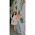WRONG OUTPUT

1.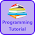Hi Pooja, what's wrong in it.

2.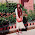I think the output is correct.

3.I have output as enter the string by user and then reverse that string

2.hi can anyone please explain fibonacci search

1.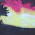http://www.cprogrammingcode.com/2014/06/program-to-print-fibonacci-series-using.html

3.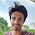for loop for push should have i<=len
i.e for(i=0;i<=len;i++){
push(str[i]);
}

1.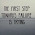Array a[n] consists of elements from a to a[n-1].So no need of taking '=' sign in the 'for' loop.

4.This comment has been removed by a blog administrator.

5.can i get the explaination of this codding??

1.Stack is a data structure in which an element that pushed last can be the first element to be popped. So, In this program we pushed all character of a string a stack and then we popped it.

2.thank you so much

6.In this program we are taking stack array as int data type.but it must be character data type.

7.In this program we are taking stack array as int data type.but it must be character data type.

8.In this program we are taking stack array as int data type.but it must be character data type.

9.In this we are taking the array stack as integer data type but it must be character datatype

10.This comment has been removed by a blog administrator.

11.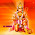can anyone explain what value will be assign to top variable initially??

1.For Stack data structure, by default top variable always point to last pushed value in stack .

12.can anyone pls explain what value should assign for top variable initially?